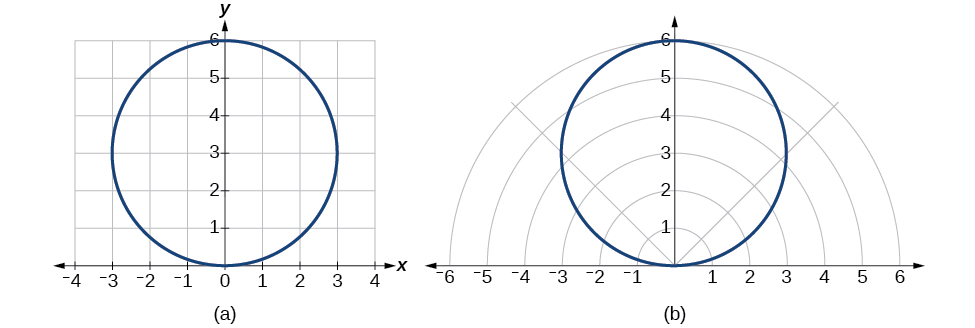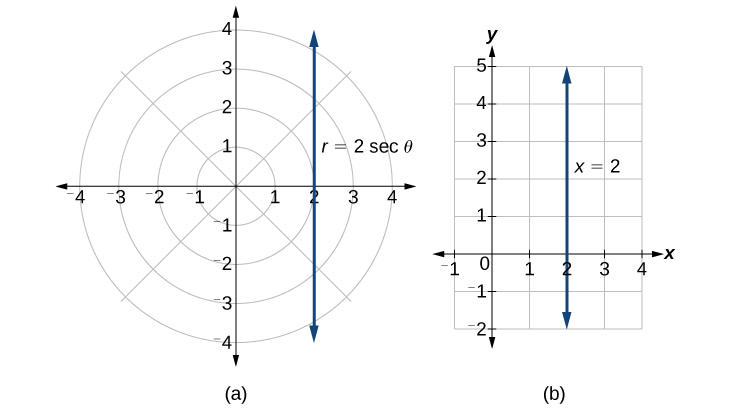# 10.3 Polar coordinates  (Page 3/8)

 Page 3 / 8

## Writing a cartesian equation in polar form

Write the Cartesian equation $\text{\hspace{0.17em}}{x}^{2}+{y}^{2}=9\text{\hspace{0.17em}}$ in polar form.

The goal is to eliminate $\text{\hspace{0.17em}}x\text{\hspace{0.17em}}$ and $\text{\hspace{0.17em}}y\text{\hspace{0.17em}}$ from the equation and introduce $\text{\hspace{0.17em}}r\text{\hspace{0.17em}}$ and $\text{\hspace{0.17em}}\theta .\text{\hspace{0.17em}}$ Ideally, we would write the equation $\text{\hspace{0.17em}}r\text{\hspace{0.17em}}$ as a function of $\text{\hspace{0.17em}}\theta .\text{\hspace{0.17em}}$ To obtain the polar form, we will use the relationships between $\text{\hspace{0.17em}}\left(x,y\right)\text{\hspace{0.17em}}$ and $\text{\hspace{0.17em}}\left(r,\theta \right).\text{\hspace{0.17em}}$ Since $\text{\hspace{0.17em}}x=r\mathrm{cos}\text{\hspace{0.17em}}\theta \text{\hspace{0.17em}}$ and $\text{\hspace{0.17em}}y=r\mathrm{sin}\text{\hspace{0.17em}}\theta ,\text{\hspace{0.17em}}$ we can substitute and solve for $\text{\hspace{0.17em}}r.$

Thus, $\text{\hspace{0.17em}}{x}^{2}+{y}^{2}=9,r=3,$ and $\text{\hspace{0.17em}}r=-3\text{\hspace{0.17em}}$ should generate the same graph. See [link] .(a) Cartesian form   x 2 + y 2 = 9   (b) Polar form   r = 3

To graph a circle in rectangular form, we must first solve for $\text{\hspace{0.17em}}y.$

Note that this is two separate functions, since a circle fails the vertical line test. Therefore, we need to enter the positive and negative square roots into the calculator separately, as two equations in the form $\text{\hspace{0.17em}}{Y}_{1}=\sqrt{9-{x}^{2}}\text{\hspace{0.17em}}$ and $\text{\hspace{0.17em}}{Y}_{2}=-\sqrt{9-{x}^{2}}.\text{\hspace{0.17em}}$ Press GRAPH.

## Rewriting a cartesian equation as a polar equation

Rewrite the Cartesian equation $\text{\hspace{0.17em}}{x}^{2}+{y}^{2}=6y\text{\hspace{0.17em}}$ as a polar equation.

This equation appears similar to the previous example, but it requires different steps to convert the equation.

We can still follow the same procedures we have already learned and make the following substitutions:

Therefore, the equations $\text{\hspace{0.17em}}{x}^{2}+{y}^{2}=6y\text{\hspace{0.17em}}$ and $\text{\hspace{0.17em}}r=6\mathrm{sin}\text{\hspace{0.17em}}\theta \text{\hspace{0.17em}}$ should give us the same graph. See [link] .(a) Cartesian form   x 2 + y 2 = 6 y (b) polar form   r = 6 sin   θ

The Cartesian or rectangular equation is plotted on the rectangular grid, and the polar equation is plotted on the polar grid. Clearly, the graphs are identical.

## Rewriting a cartesian equation in polar form

Rewrite the Cartesian equation $\text{\hspace{0.17em}}y=3x+2\text{\hspace{0.17em}}$ as a polar equation.

We will use the relationships $\text{\hspace{0.17em}}x=r\mathrm{cos}\text{\hspace{0.17em}}\theta \text{\hspace{0.17em}}$ and $\text{\hspace{0.17em}}y=r\mathrm{sin}\text{\hspace{0.17em}}\theta .$

Rewrite the Cartesian equation $\text{\hspace{0.17em}}{y}^{2}=3-{x}^{2}\text{\hspace{0.17em}}$ in polar form.

$r=\sqrt{3}$

## Identify and graph polar equations by converting to rectangular equations

We have learned how to convert rectangular coordinates to polar coordinates, and we have seen that the points are indeed the same. We have also transformed polar equations to rectangular equations and vice versa. Now we will demonstrate that their graphs, while drawn on different grids, are identical.

## Graphing a polar equation by converting to a rectangular equation

Covert the polar equation $\text{\hspace{0.17em}}r=2\mathrm{sec}\text{\hspace{0.17em}}\theta \text{\hspace{0.17em}}$ to a rectangular equation, and draw its corresponding graph.

The conversion is

Notice that the equation $\text{\hspace{0.17em}}r=2\mathrm{sec}\text{\hspace{0.17em}}\theta \text{\hspace{0.17em}}$ drawn on the polar grid is clearly the same as the vertical line $\text{\hspace{0.17em}}x=2\text{\hspace{0.17em}}$ drawn on the rectangular grid (see [link] ). Just as $\text{\hspace{0.17em}}x=c\text{\hspace{0.17em}}$ is the standard form for a vertical line in rectangular form, $\text{\hspace{0.17em}}r=c\mathrm{sec}\text{\hspace{0.17em}}\theta \text{\hspace{0.17em}}$ is the standard form for a vertical line in polar form.(a) Polar grid (b) Rectangular coordinate system

A similar discussion would demonstrate that the graph of the function $\text{\hspace{0.17em}}r=2\mathrm{csc}\text{\hspace{0.17em}}\theta \text{\hspace{0.17em}}$ will be the horizontal line $\text{\hspace{0.17em}}y=2.\text{\hspace{0.17em}}$ In fact, $\text{\hspace{0.17em}}r=c\mathrm{csc}\text{\hspace{0.17em}}\theta \text{\hspace{0.17em}}$ is the standard form for a horizontal line in polar form, corresponding to the rectangular form $\text{\hspace{0.17em}}y=c.$

#### Questions & Answers

x exposant 4 + 4 x exposant 3 + 8 exposant 2 + 4 x + 1 = 0
x exposent4+4x exposent3+8x exposent2+4x+1=0
HERVE
How can I solve for a domain and a codomains in a given function?
ranges
EDWIN
Thank you I mean range sir.
Oliver
proof for set theory
don't you know?
Inkoom
find to nearest one decimal place of centimeter the length of an arc of circle of radius length 12.5cm and subtending of centeral angle 1.6rad
factoring polynomial
find general solution of the Tanx=-1/root3,secx=2/root3
find general solution of the following equation
Nani
the value of 2 sin square 60 Cos 60
0.75
Lynne
0.75
Inkoom
when can I use sin, cos tan in a giving question
depending on the question
Nicholas
I am a carpenter and I have to cut and assemble a conventional roof line for a new home. The dimensions are: width 30'6" length 40'6". I want a 6 and 12 pitch. The roof is a full hip construction. Give me the L,W and height of rafters for the hip, hip jacks also the length of common jacks.
John
I want to learn the calculations
where can I get indices
I need matrices
Nasasira
hi
Raihany
Hi
Solomon
need help
Raihany
maybe provide us videos
Nasasira
Raihany
Hello
Cromwell
a
Amie
What do you mean by a
Cromwell
nothing. I accidentally press it
Amie
you guys know any app with matrices?
Khay
Ok
Cromwell
Solve the x? x=18+(24-3)=72
x-39=72 x=111
Suraj
Solve the formula for the indicated variable P=b+4a+2c, for b
Need help with this question please
b=-4ac-2c+P
Denisse
b=p-4a-2c
Suddhen
b= p - 4a - 2c
Snr
p=2(2a+C)+b
Suraj
b=p-2(2a+c)
Tapiwa
P=4a+b+2C
COLEMAN
b=P-4a-2c
COLEMAN
like Deadra, show me the step by step order of operation to alive for b
John
A laser rangefinder is locked on a comet approaching Earth. The distance g(x), in kilometers, of the comet after x days, for x in the interval 0 to 30 days, is given by g(x)=250,000csc(π30x). Graph g(x) on the interval [0, 35]. Evaluate g(5)  and interpret the information. What is the minimum distance between the comet and Earth? When does this occur? To which constant in the equation does this correspond? Find and discuss the meaning of any vertical asymptotes.
The sequence is {1,-1,1-1.....} hasByByByBy Sam Luong